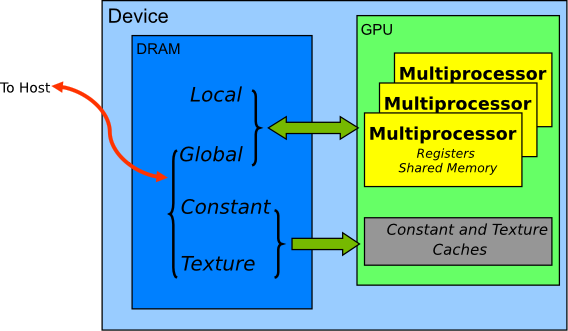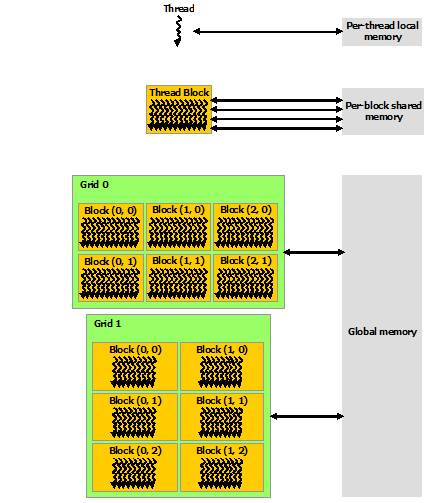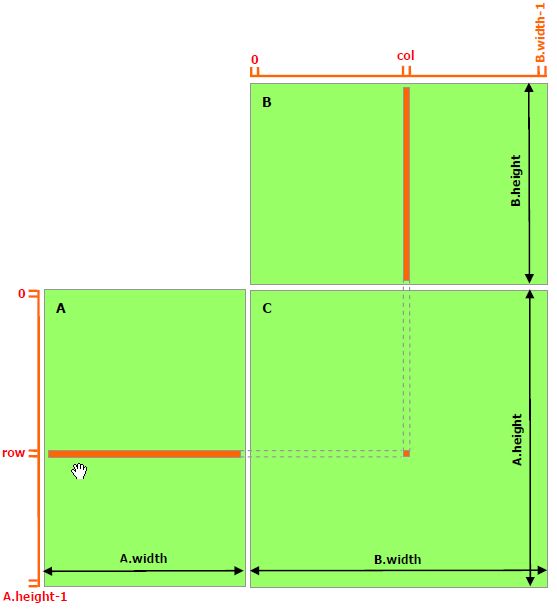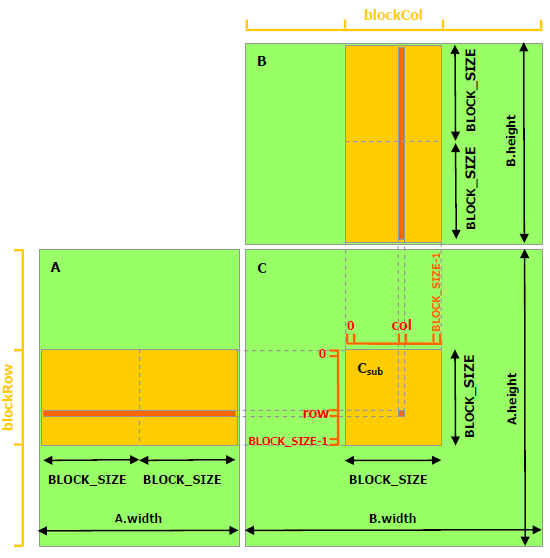# Shared MemoryGPU内存结构

GPU的内存结构如图所示：GPU的计算核心都在Streaming Multiprocessor（SM）上，SM里有计算核心可直接访问的寄存器（Register）和共享内存（Shared Memory）；多个SM可以读取显卡上的显存，包括全局内存（Global Memory）。每个SM上的Shared Memory相当于该SM上的一个缓存，一般都很小，Telsa V100的Shared Memory也只有96KB。注意，Shared Memory和Global Memory的字面上都有共享的意思，但是不要将两者的概念混淆，Shared Memory离计算核心更近，延迟很低；Global Memory是整个显卡上的全局内存，延迟高。计算与存储之间的关系

## 普通矩阵乘法#from numba import cudaimport numpy as npimport mathfrom time import time
@cuda.jitdef matmul(A, B, C):    """  矩阵乘法 C = A * B    """    # Numba库提供了更简易的计算方法    # x, y = cuda.grid(2)    # 具体计算公式如下    row = cuda.threadIdx.x + cuda.blockDim.x * cuda.blockIdx.x    col = cuda.threadIdx.y + cuda.blockDim.y * cuda.blockIdx.y            if row < C.shape and col < C.shape:        tmp = 0.        for k in range(A.shape):            tmp += A[row, k] * B[k, col]        C[row, col] = tmp        def main():    # 初始化矩阵    M = 6000    N = 4800    P = 4000    A = np.random.random((M, N)) # 随机生成的 [M x N] 矩阵    B = np.random.random((N, P)) # 随机生成的 [N x P] 矩阵        start = time()    A = cuda.to_device(A)    B = cuda.to_device(B)    C_gpu = cuda.device_array((M, P))
# 执行配置    threads_per_block = (16, 16)    blocks_per_grid_x = int(math.ceil(A.shape / threads_per_block))    blocks_per_grid_y = int(math.ceil(B.shape / threads_per_block))    blocksPerGrid = (blocks_per_grid_x, blocks_per_grid_y)        # 启动核函数    matmul[blocksPerGrid, threads_per_block](A, B, C_gpu)
# 数据拷贝    C = C_gpu.copy_to_host()    cuda.synchronize()
print("gpu matmul time :" + str(time() - start))
start = time()    C_cpu = np.empty((M, P), np.float)    np.matmul(A, B, C_cpu)    print("cpu matmul time :" + str(time() - start))
# 验证正确性    if np.allclose(C_cpu, C):        print("gpu result correct")
if __name__ == "__main__":    main()

## 基于Shared Memory的矩阵乘法#from numba import cuda, float32import numpy as npimport mathfrom time import time
# thread per block# 每个block有 BLOCK_SIZE x BLOCK_SIZE 个元素BLOCK_SIZE = 16
@cuda.jitdef matmul(A, B, C):    """  矩阵乘法 C = A * B    """    row = cuda.threadIdx.x + cuda.blockDim.x * cuda.blockIdx.x    col = cuda.threadIdx.y + cuda.blockDim.y * cuda.blockIdx.y        if row < C.shape and col < C.shape:        tmp = 0.        for k in range(A.shape):            tmp += A[row, k] * B[k, col]        C[row, col] = tmp
@cuda.jitdef matmul_shared_memory(A, B, C):    """    使用Shared Memory的矩阵乘法 C = A * B    """    # 在Shared Memory中定义向量    # 向量可被整个Block的所有Thread共享    # 必须声明向量大小和数据类型    sA = cuda.shared.array(shape=(BLOCK_SIZE, BLOCK_SIZE), dtype=float32)    sB = cuda.shared.array(shape=(BLOCK_SIZE, BLOCK_SIZE), dtype=float32)        tx = cuda.threadIdx.x    ty = cuda.threadIdx.y    row = cuda.threadIdx.x + cuda.blockDim.x * cuda.blockIdx.x    col = cuda.threadIdx.y + cuda.blockDim.y * cuda.blockIdx.y        if row >= C.shape and col >= C.shape:        # 当(x, y)越界时退出        return
tmp = 0.    # 以一个 BLOCK_SIZE x BLOCK_SIZE 为单位    for m in range(math.ceil(A.shape / BLOCK_SIZE)):        sA[tx, ty] = A[row, ty + m * BLOCK_SIZE]        sB[tx, ty] = B[tx + m * BLOCK_SIZE, col]        # 线程同步，等待Block中所有Thread预加载结束        # 该函数会等待所有Thread执行完之后才执行下一步        cuda.syncthreads()        # 此时已经将A和B的子矩阵拷贝到了sA和sB
# 计算Shared Memory中的向量点积        # 直接从Shard Memory中读取数据的延迟很低        for n in range(BLOCK_SIZE):            tmp += sA[tx, n] * sB[n, ty]
# 循环后得到每个BLOCK的点积之和    C[row, col] = tmp
def main():    # 初始化矩阵    M = 6000    N = 4800    P = 4000    A = np.random.random((M, N)) # 随机生成的 [M x N] 矩阵    B = np.random.random((N, P)) # 随机生成的 [N x P] 矩阵
A_device = cuda.to_device(A)    B_device = cuda.to_device(B)    C_device = cuda.device_array((M, P)) # [M x P] 矩阵
# 执行配置    threads_per_block = (BLOCK_SIZE, BLOCK_SIZE)    blocks_per_grid_x = int(math.ceil(A.shape / BLOCK_SIZE))    blocks_per_grid_y = int(math.ceil(B.shape / BLOCK_SIZE))    blocks_per_grid = (blocks_per_grid_x, blocks_per_grid_y)
start = time()    matmul[blocks_per_grid, threads_per_block](A_device, B_device, C_device)    cuda.synchronize()    print("matmul time :" + str(time() - start))
start = time()    matmul_shared_memory[blocks_per_grid, threads_per_block](A_device, B_device, C_device)    cuda.synchronize()    print("matmul with shared memory time :" + str(time() - start))    C = C_device.copy_to_host()
if __name__ == "__main__":    main()

matmul time :1.4370720386505127matmul with shared memory time :0.7994928359985352

1. 声明Shared Memory。这里使用了cuda.shared.array(shape,type)shape为这块数据的向量维度大小，type为Numba数据类型，例如是int32还是float32。这个函数只能在设备端使用。定义好后，这块数据可被同一个Block的所有Thread共享。需要注意的是，这块数据虽然在核函数中定义，但它不是单个Thread的私有数据，它可被同Block中的所有Thread读写

2. 数据加载。每个Thread会将A中的一个元素加载到sA中，一个Block的 BLOCK_SIZE x BLOCK_SIZE 个Thread可以把sA填充满。cuda.syncthreads()会等待Block中所有Thread执行完之后才执行下一步。所以，当执行完这个函数的时候，sA和sB的数据已经拷贝好了。

3. 数据复用。A中的某个点，只会被读取 B.width / BLOCK_SIZE 次；B中的某个点，只会被读 A.height / BLOCK_SIZE 次。for n in range(BLOCK_SIZE)这个循环做子矩阵向量乘法时，可多次复用sA和sB的数据。

4. 子矩阵的数据汇总。我们以一个 BLOCK_SIZE x BLOCK_SIZE 的子矩阵为单位分别对A从左到右，对B从上到下平移并计算，共循环 A.width / BLOCK_SIZE 次。在某一步平移，会得到子矩阵的点积。for m in range(math.ceil(A.shape / BLOCK_SIZE))这个循环起到了计算A从左到右与B从上到下点积的过程。